NCERT Solutions for Class 10 Mathematics Chapter 3 Pair of Linear Equations in Two Variables Ex 3.7 are part of NCERT Solutions for Class 10 Maths. Here we have given NCERT Solutions for Class 10 Mathematics Chapter 3 Pair of Linear Equations in Two Variables Ex 3.7.

 Board CBSE Textbook NCERT Class Class 10 Subject Maths Chapter Chapter 3 Chapter Name Pair of Linear Equations in Two Variables Exercise Ex 3.7 Number of Questions Solved 8 Category NCERT Solutions

### NCERT Solutions for Class 10 Maths Chapter 3 Pair of Linear Equations in Two Variables Ex 3.7

Question 1.
The age of two friends Ani and Biju differ by 3 years. Ani’s father Dharam is twice as old as Ani and Biju is twice as old as his sister Cathy. The ages of Cathy and Dharam differ by 30 years. Find the ages of Ani and Biju.
Solution:
Let the ages of Ani and Biju be x years and y years respectively.
If Ani is older than Biju
x – y =3
If Biju is older than Ani
y – x = 3
-x + y =3   [Given]Subtracting equation (i) from equation (ii), we get:
3x – 57
⇒ x = 19
Putting x = 19 in equation (i), we get
19-y = 3
⇒ y = 16
Again subtracting equation (iv) from equation (iii), we get
3x = 63
⇒  x =  21
Putting x = 21 in equation (iii) we get
21 -y=  -3
⇒  y  =   24
Hence, Ani’s age is   either 19 years or 21 years and Biju’s age is either 16 years or 24 years.

Question 2.
One says, “Give me a hundred, friend! I shall then become twice as rich as you”. The other replies, “If you give me ten, I shall be six times as rich as you”. Tell me what is the amount of their (respective) capital?
Solution:
Let the two friends have ₹ x and ₹ y.
According to the first condition:
One friend has an amount = ₹(x + 100)
Other has an amount = ₹ (y – 100
∴  (x + 100) =2 (y – 100)
⇒  x + 100 = 2y – 200
⇒ x – 2y = -300       …(i)
According to the second condition:
One friend has an amount = ₹(x – 10)
Other friend has an amount =₹ (y + 10)
∴  6(x – 10) = y + 10
⇒ 6x – 60 = y + 10
⇒    6x-y = 70                                        …(ii)
Multiplying (ii) equation by 2 and subtracting the result from equation (i), we get:
x – 12x = – 300 – 140
⇒ -11x = -440
⇒  x = 40
Substituting x = 40 in equation (ii), we get
6 x 40 – y = 70
⇒ -y   = 70- 24
⇒  y   = 170
Thus, the two friends have ₹ 40 and ₹ 170.

Question 3.
A train covered a certain distance at a uniform speed. If the train would have been 10 km/h faster, it would have taken 2 hours less than the scheduled time. And, if the train were slower by 10 km/h, it would have taken 3 hours more than the scheduled time. Find the distance covered by the train.
Solution:
Let the original speed of the train be x km/h
and the time taken to complete the journey be y hours.            ‘
Then the distance covered = xy km

Case I: When speed = (x + 10) km/h and time taken = (y – 2) h
Distance = (x + 10) (y – 2) km
⇒   xy = (x + 10) (y – 2)
⇒ 10y – 2x = 20
⇒  5y – x = 10
⇒ -x + 5y = 10   …(i)

Case II: When speed = (x – 10) km/h and time taken = (y + 3) h
Distance = (x – 10) (y + 3) km
⇒  xy = (x – 10) (y + 3)
⇒ 3x- 10y = 30    …(ii)
Multiplying equation (i) by 3 and adding the result to equation (ii), we get
15y – 10y = 30 f 30
⇒ 5y = 60
⇒   y   = 12
Putting y = 12 in equation (ii), we get
3x- 10 x 12= 30
⇒  3x   = 150
⇒ x   = 50
∴  x = 50 and y =   12
Thus, original speed of train is 50 km/h and time taken by it is 12 h.
Distance covered by train = Speed x Time
=  50 x 12 = 600 km.

Question 4.
The students of a class are made to stand in rows. If 3 students are extra in a row, there would be 1 row less. If 3 students are less in a row, there would be 2 rows more. Find the number of students in the class.
Solution:
Let the number of rows be x and the number of students in each row be y.
Then the total number of students = xy
Case I: When there are 3 more students in each row
Then the number of students in a row = (y + 3)
and the number of rows = (x – 1)
Total number of students = (x – 1) (y + 3)
∴ (x – 1) (y + 3) = xy
⇒  3x  -y =3 …(i)
Case II: When 3 students are removed from each row
Then the number of students in each row = (y-3)
and the number of rows = (x + 2)
Total number of students = (x + 2) (y – 3)
∴  (x + 2) (y – 3) = xy
⇒ -3x + 2y = 6 …(ii)
Adding the equations (i) and (ii), we get
-y + 2y = 3 + 6
⇒ y = 9
Putting y = 9 in the equation (ii), we get
-3x +   18 = 6
⇒ x = 4
∴ x = 4 and y = 9
Hence, the total number of students in the class is 9 x 4 = 36.

Question 5.
In a ∆ABC, ∠C = 3 ∠B = 2(∠A + ∠B). Find the three angles.
Solution:
Let ∠A = x° and ∠B = y°.
Then ∠C = 3∠B = (3y)°.
Now ∠A + ∠B + ∠C = 180°
⇒ x + y + 3y = 180°
⇒ x + 4y = 180° …(i)
Also, ∠C = 2(∠A + ∠B)
⇒ 3y – 2(x + y)
⇒ 2x – y = 0° …(ii)
Multiplying (ii) by 4 and adding the result to equation (i), we get:
9x = 180°
⇒ x = 20°
Putting x = 20 in equation (i), we get:
20 + 4y = 180°
⇒ 4y = 160°
⇒  y =  $$\frac { 160 }{ 40 }$$  = 40°
∴ ∠A = 20°, ∠B = 40° and ∠C = 3 x 40° = 120°.

Question 6.
Draw the graphs of the equations 5x – y = 5 and 3x – y = 3. Determine the coordinates of the vertices of the triangle formed by these lines and the y-axis.
Solution:
5x – y = 5    …(i)
3x-y = 3    …(ii)
For graphical representation:
From equation (i), we get: y = 5x – 5
When x = 0, then y -5
When x = 2, then y = 10 – 5 = 5
When x = 1, then y = 5 – 5 = 10
Thus, we have the following table of solutions:From equation (ii), we get:
⇒ y = 3x – 3
When x = 0, then y = -3
When x = 2, then y = 6 – 3 = 3
When x = 1, then y = 3 – 3 = 0
Thus, we have the following table of solutions: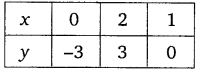Plotting the points of each table of solutions, we obtain the graphs of two lines intersecting each other at a point C(1, 0).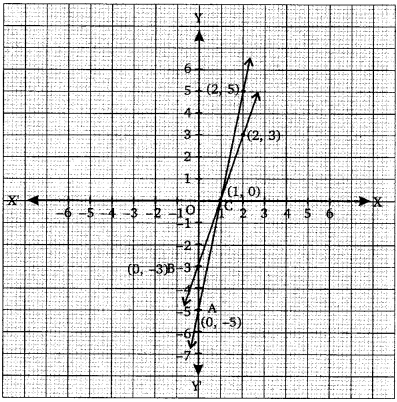The vertices of ΔABC formed by these lines and the y-axis are A(0, -5), B(0, -3) and C(1, 0).

Question 7.
Solve the following pairs of linear equations: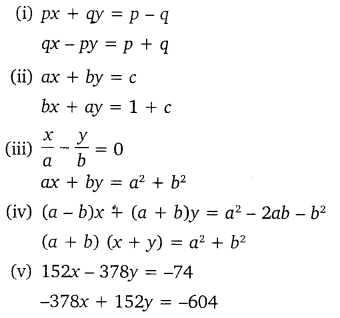Solution:
(i) The given equations are
px + qy = p – q  …(1)
qx – py = p + q …(2)
Multiplying equation (1) byp and equation (2) by q and then adding the results, we get:
x(p2 + q2) = p(p – q) + q(p + q)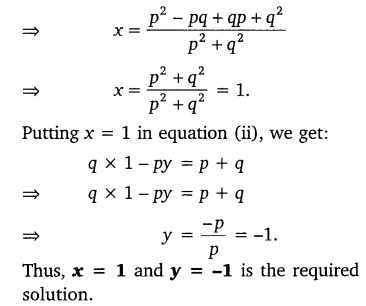(ii) The given equations are
ax + by = c  …(1)
bx – ay = 1 + c       …(2)
Multiplying equation (1) by b and equation (2) by a, we get:
abx + b2y = cb …(3)
abx + a2y = a(1+ c)  …(4)
Subtracting (3) from (4), we get:(iii) The given equations may be written as: bx – ay = 0  …(1)
ax + by = a2 + b2   …(2)
Multiplying equation (1) by b and equation (2) by a, we get:
b2x + aby = 0 ….(3)
a2x + aby = a(a2 + b2) …..(4)
Adding equation (3) and equation (4), we get:
(a2 + b2)x = a (a2 + b2) a(a2 + b2)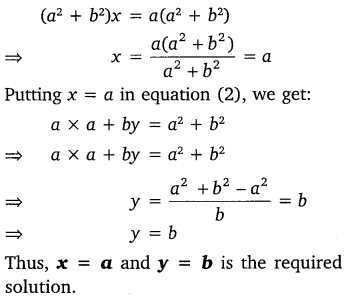(iv) The given equations may be written as:
(a – b)x + (a + b)y = a2 – 2ab – b2 …(1)
(a + b)x + (a + b)y = a2 + b2 …(2)
Subtracting equation (2) from equation (1), we get:
(a – b)x – (a + b)x
= (a2 – 2ab – b2) – (a2 + b2)
⇒ x(a – b- a-b) = a2 – 2ab – b2 – a2 – b2
⇒   -2bx = -2ab – 2b2
⇒ 2bx = 2b2 + 2ab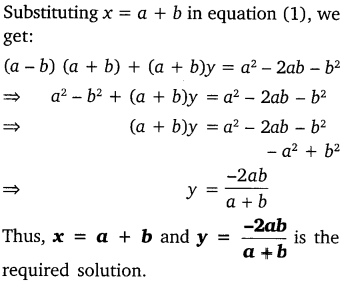(v) The given equations may be written as:
76x – 189y = -37 …(1)
-189x + 76y = -302   …(2)
Multiplying equation (1) by 76 and equation (2) by 189, we get:
5776x – 14364y = -2812  …(3)
-35721x + 14364y = -57078 …(4)
Adding equations (3) and (4), we get:
5776x – 35721x = -2812 – 57078
⇒ – 29945x = -59890
⇒  x = 2
Putting x = 2 in equation (1), we get:
76   x  2 – 189y   = -37
⇒ 152 – 189y   = -37
⇒ -189y  = -189
⇒  y = 1
Thus, x = 2 and y = 1 is the required solution.

Question 8.
ABCD is a cyclic quadrilateral (see figure). Find the angles of the cyclic quadrilateral.Solution:We hope the NCERT Solutions for Class 10 Mathematics Chapter 3 Pair of Linear Equations in Two Variables Ex 3.7 help you. If you have any query regarding NCERT Solutions for Class 10 Mathematics Chapter 3 Pair of Linear Equations in Two Variables Ex 3.7, drop a comment below and we will get back to you at the earliest.